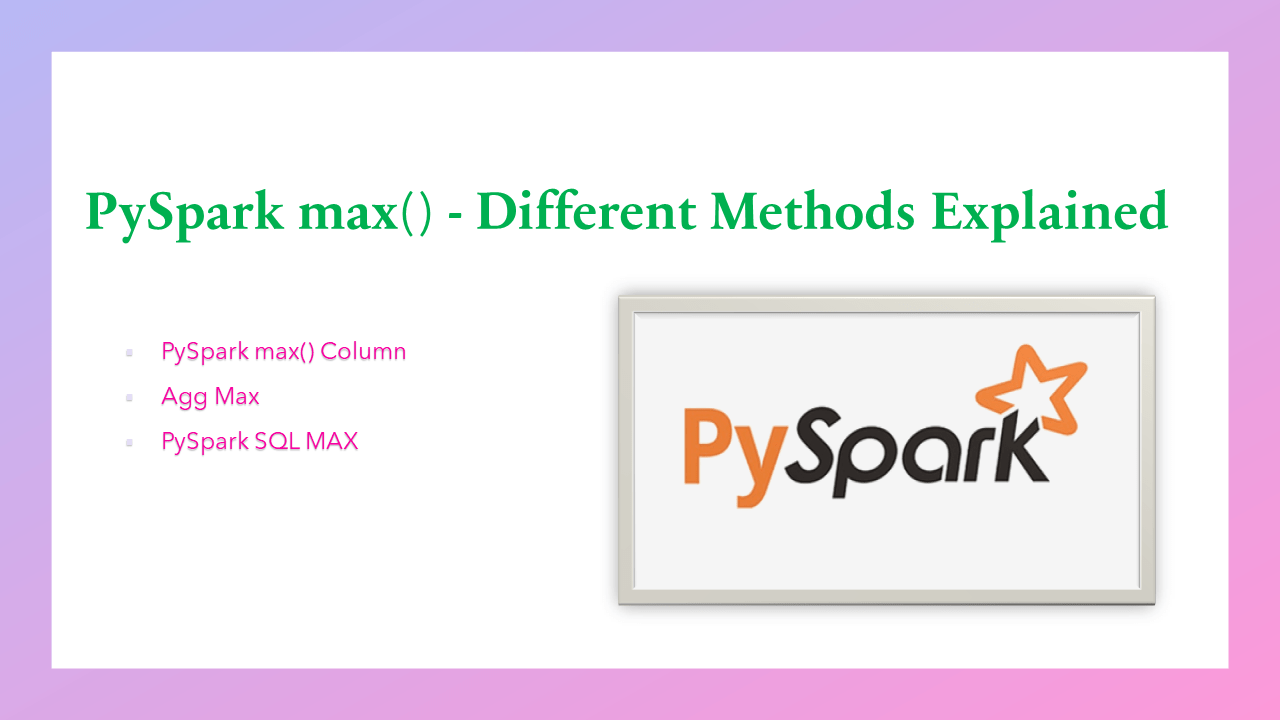# PySpark max() – Different Methods Explained

PySpark max() function is used to get the maximum value of a column or get the maximum value for each group. PySpark has several max() functions, depending on the use case you need to choose which one fits your need.

Let’s create a PySpark DataFrame and use these functions to get the max value of single or multiple columns.

``````
# Imports
from pyspark.sql import SparkSession

# Create SparkSession
spark = SparkSession.builder \
.appName('SparkByExamples.com') \
.getOrCreate()

# Prepare Data
simpleData = (("Java",4000,5), \
("Python", 4600,10),  \
("Scala", 4100,15),   \
("Scala", 4500,15),   \
("PHP", 3000,20),  \
)
columns= ["CourseName", "fee", "discount"]

# Create DataFrame
df = spark.createDataFrame(data = simpleData, schema = columns)
df.printSchema()
df.show(truncate=False)
``````

Yields below output.

## 3. PySpark max() Column

`pyspark.sql.functions.max()` is used to get the maximum value of a column. By using this we can perform a max of a single column and a max of multiple columns of DataFrame. While performing the max it ignores the null/none values from the column. In the below example,

• DataFrame.select() is used to get the DataFrame with the selected columns.
• df.fee refers to the name column of the DataFrame.
• max(df.fee) get the maximum of a column.
``````
# Using max() function
from pyspark.sql.functions import max
df.select(max(df.fee)).show()

# Using max() on multiple columns
from pyspark.sql.functions import max
df.select(max(df.fee).alias("fee_max"),
max(df.discount).alias("discount_max")
).show()
``````

Yields below output.

## 5. GroupedData.max()

`GroupedData.max()` is used to get the max for each group. In the below example, DataFrame.groupBy() is used to perform the grouping on `coursename` column and returns a GroupedData object. When you perform group by, the data having the same key are shuffled and brought together. Since it involves the data crawling across the network, group by is considered a wider transformation.

Now perform `GroupedData.max()` to get the max for each course.

``````
# groupby max on all columns
df.groupBy("CourseName").max() \
.show()

# groupby max on selected column
df.groupBy("CourseName").max("fee") \
.show()
``````

Yields below output.

## 6. Agg Max

Use the DataFrame.agg() function to get the max from the column in the dataframe. This method is known as aggregation, which allows to group the values within a column or multiple columns. It takes the parameter as a dictionary with the key being the column name and the value being the aggregate function (sum, count, min, max e.t.c).

``````
# Using agg max
df.agg({'discount':'max','fee':'max'}).show()
``````

## 7. PySpark SQL MAX

In PySpark SQL, you can use max(column_name) to get the max of DataFrame column. In order to use SQL, make sure you create a temporary view using createOrReplaceTempView().

To run the SQL query use `spark.sql()` function and create the table by using createOrReplaceTempView(). This table would be available to use until you end your current SparkSession.

`spark.sql()` returns a DataFrame and here, I have used show() to display the contents to console.

``````
# PySpark SQL MAX
df.createOrReplaceTempView("COURSE")
spark.sql("SELECT MAX(FEE) FROM COURSE").show()
spark.sql("SELECT MAX(FEE), MAX(DISCOUNT) FROM COURSE").show()
spark.sql("SELECT COURSENAME,MAX(FEE) FROM COURSE GROUP BY COURSENAME").show()
``````

## Complete Example of PySpark Max

Following is the complete example of PySpark max with all the different functions.

``````
# Imports
from pyspark.sql import SparkSession

# Create SparkSession
spark = SparkSession.builder \
.appName('SparkByExamples.com') \
.getOrCreate()

# Prepare Data
simpleData = (("Java",4000,5), \
("Python", 4600,10),  \
("Scala", 4100,15),   \
("Scala", 4500,15),   \
("PHP", 3000,20),  \
)
columns= ["CourseName", "fee", "discount"]

# Create DataFrame
df = spark.createDataFrame(data = simpleData, schema = columns)
df.printSchema()
df.show(truncate=False)

# Using max() function
from pyspark.sql.functions import max
df.select(max(df.fee)).show()

# Using max() on multiple columns
from pyspark.sql.functions import max
df.select(max(df.fee).alias("fee_max"),
max(df.discount).alias("discount_max")
).show()

# groupby max on all columns
df.groupBy("CourseName").max() \
.show()

# groupby max on selected column
df.groupBy("CourseName").max("fee") \
.show()

# Using agg max
df.agg({'discount':'max','fee':'max'}).show()

df.createOrReplaceTempView("Course")
df2 = spark.sql("select coursename, max(fee) fee_max, max(discount) discount_max " \
"from course group by coursename")
df2.show()

# Imports to use Pandas API
import pyspark.pandas as ps
import numpy as np

technologies = ({
'Courses':["Spark",np.nan,"pandas","Java","Spark"],
'Fee' :[20000,25000,30000,22000,np.NaN],
'Duration':['30days','40days','35days','60days','50days'],
'Discount':[1000,2500,1500,1200,3000]
})
df = ps.DataFrame(technologies)

print(df)
print(df.max())
``````

## 8. Conclusion

In this article, you have learned different ways to get the max value of a column in PySpark DataFrame. By using functions.max(), GroupedData.max() you can get the max of a column, each of these functions is used for a different purpose. Also, you can use ANSI SQL to get the max.

## References

### Naveen (NNK)

Naveen (NNK) is a Data Engineer with 20+ years of experience in transforming data into actionable insights. Over the years, He has honed his expertise in designing, implementing, and maintaining data pipelines with frameworks like Apache Spark, PySpark, Pandas, R, Hive and Machine Learning. Naveen journey in the field of data engineering has been a continuous learning, innovation, and a strong commitment to data integrity. In this blog, he shares his experiences with the data as he come across. Follow Naveen @ @ LinkedIn